## Eigenfunctions, Eigenvalues and Vector Spaces

For any given physical problem, the Schrödinger equation solutions which separate (between time and space),are an extremely important set. If we assume the equation separates, we get the two equations (in one dimension for simplicity)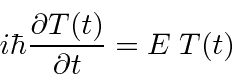The second equation is called the time independent Schrödinger equation. For bound states, there are only solutions to that equation for some quantized set of energiesFor states which are not bound, a continuous range of energies is allowed.

The time independent Schrödinger equation is an example of an eigenvalue equation.If we operate on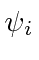with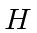, we get back the same functiontimes some constant. In this casewould be called and Eigenfunction, and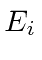would be called an Eigenvalue. There are usually an infinite number of solutions, indicated by the indexhere.

Operators for physical variables must have real eigenvalues. They are called Hermitian operators. We can show that the eigenfunctions of Hermitian operators are orthogonal (and can be normalized).(In the case of eigenfunctions with the same eigenvalue, called degenerate eigenfunctions, we can must choose linear combinations which are orthogonal to each other.) We will assume that the eigenfunctions also form a complete set so that any wavefunction can be expanded in them,where the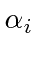are coefficients which can be easily computed (due to orthonormality) bySo now we have another way to represent a state (in addition to position space and momentum space). We can represent a state by giving the coefficients in sum above. (Note thatis just an eigenfunction of the momentum operator andis just an eigenfunction of the position operator (in p-space) so they also represent and expansion of the state in terms of eigenfunctions.)

Since theform an orthonormal, complete set, they can be thought of as the unit vectors of a vector space. The arbitrary wavefunctionwould then be a vector in that space and could be represented by its coefficients.The bra-ketcan be thought of as a dot product between the arbitrary vectorand one of the unit vectors. We can use the expansion in terms of energy eigenstates to compute many things. In particular, since the time development of the energy eigenstates is very simple,we can use these eigenstates to follow the time development of an arbitrary statesimply by computing the coefficientsat.

We can define the Hermitian conjugate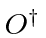of the operatorby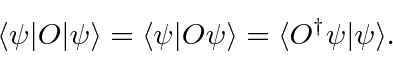Hermitian operatorshave the property that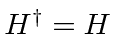.

Jim Branson 2013-04-22